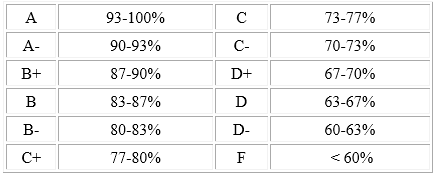# Midterm 1 and Current Course Grades in Both Sections of Finance 4335

I just uploaded the midterm 1 grades, along with attendance, quiz, problem set, and current Finance 4335 course grades to Canvas.

As stated in the course syllabus, final numeric course grades will be determined according to the following equation:

Final Course Numeric Grade =.10(Attendance and Participation) +.10(Quizzes) +.20(Problem Sets) + Max{.20(Midterm Exam 1) +.20(Midterm Exam 2) +.20(Final Exam),.20(Midterm Exam 1) +.40(Final Exam),.20(Midterm Exam 2) +.40(Final Exam)}

As I noted in my September 2nd blog posting entitled “Finance 4335 Grades on Canvas”, as the fall semester progresses and I continue to collect grades in the attendance, quiz, problem set, and exam categories, then the course grade listed on Canvas will dynamically incorporate that information on a timely basis for each student; now that we have Midterm 1 Exam grades, the equation that I am now using (until Midterm 2) is as follows:

Course Numeric Grade after Midterm 1 = (.10(Attendance and Participation) +.10(Quizzes) +.20(Problem Sets) +.20(Midterm 1))/.6

There are n = 65 students enrolled in both sections of Finance 4335. Here are the current grade statistics (n = 64 for midterm exam 1 statistics because one student opted to not take midterm exam 1):As you can see from this table, over 50% of students have the mean or higher in each category (since in all cases, the median is higher than the mean). I base the GPA calculation on comparing each student’s current course grade to the course letter grade schedule that also appears on the syllabus:If you are disappointed by your performance so far in Finance 4335, keep in mind that the final exam grade automatically double counts in place of a lower midterm exam grade. In case if both midterm exam grades are lower than the final exam grade, then the final exam grade replaces the lower of the two midterm exam grades.  If any of you would like to have a chat with me about your grades, by all means, then set up a Zoom appointment with me.